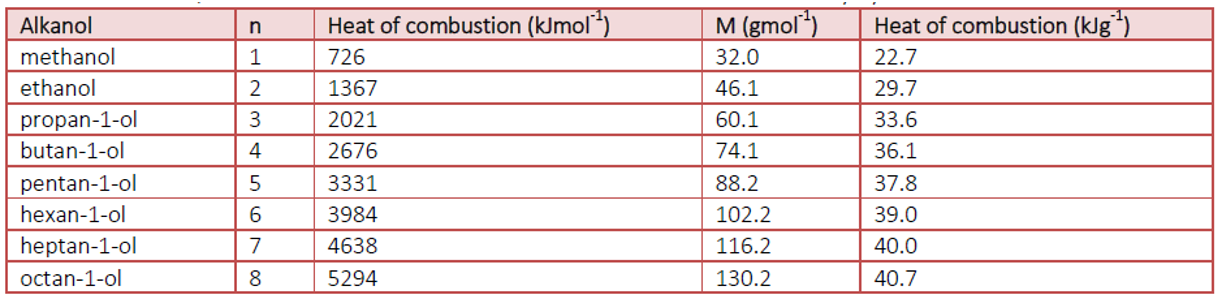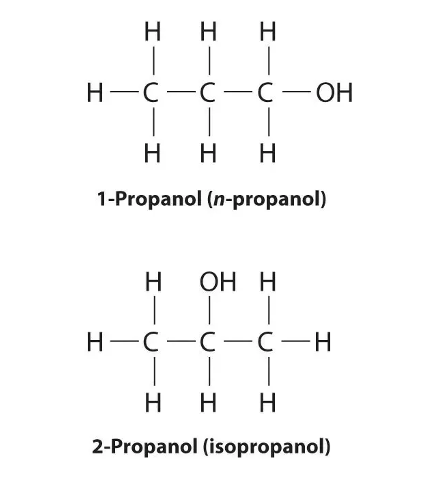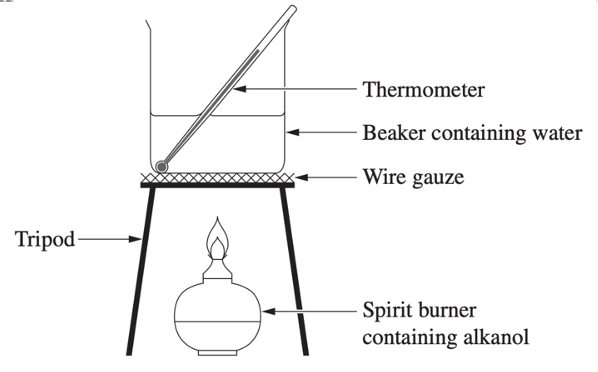# Combustion of Alcohols & Enthalpy of Combustion

This is part of the HSC Chemistry course under the topic Alcohols.

– combustion

### Measuring Enthalpy of Combustion

This video discusses how the enthalpy of combustion of various alcohols can be compared experimentally. The video also walks through calculations of enthalpy of combustion.

### Combustion of Alcohols

All alcohols undergo combustion with oxygen to produce carbon dioxide and water (complete combustion). For example, the combustion of ethanol:

$$C_2H_5OH(l) + 3O_2(g) \rightarrow 2CO_2(g) + 3H_2O(l)$$

Combustion of alcohol is exothermic which means the energy released during bond formation of products is greater than the energy absorbed during bond breaking of reactants. In alcohol combustion, all of the energy comes from the formation of C=O bonds in CO2 and H–O bonds in water.

 Bond type Energy absorbed or released (kJ mol–1) Number of extra bond broken/formed for each new carbon atom Change in enthalpy for each new carbon atom (kJ mol–1) C–C +347 1 1 × 347 = 347 C–H +413 2 2 × 413 = 826 C=O –746 2 2 × –746 = –1492 O–H –464 2 2 × –464 = –928

Molar enthalpy of alochols, ∆H (in kJ mol–1) increases with alcohol size.

For each additional carbon atom added to an alcohol molecule, two extra C–H bonds and one extra C–H bond are broken during combustion. This is followed by the formation of two extra C=O bonds in a carbon dioxide molecule and two extra O–H bonds in a water molecule. Thus, as shown in table above, adding carbon atoms to an alcohol molecule causes its combustion to produce more energy i.e. ∆H to become more negative.

Table: heat of combustion and molecular weight of first eight straight-chained alcohols.∆H (in kJ g–1) increases with alcohol size.

For each additional carbon atom added to an alcohol molecule, its molecular mass increases constantly (1 carbon atom and 2 hydrogen atoms). Since O–H bonds in alcohol molecules are not broken during combustion, mass of oxygen atom(s) (16.00 g mol–1) does not contribute to ∆H of combustion. As carbon atoms are added to increase the size of alcohol, oxygen’s contribution to molecular mass becomes smaller, leading to an increase in ∆H (in kJ g–1).

Position and chain isomers have the same heat of combustion (kJ mol–1 and kJ g–1) because the bonds broken and formed are identical despite their differences in structure. For example, 1-propanol and 2-propanol are position isomers.Number of bonds broken C–H = 7 C–C = 2 C–O = 1 Number of bonds broken C–H = 7 C–C = 2 C–O = 1

### Investigating Enthalpy of Combustion of Alcohols

• Heat of combustion (∆cH) of an alcohol is the amount of heat energy it releases per mole or gram of alcohol consumed.
• An example of a first-hand investigation to measure a 1-propanol’s heat of combustion:1. 1-propanol is added to a spirit burner and the total mass is weighed on an electronic balance.
2. A known quantity of water is added to a 500 mL beaker.
3. The initial temperature of water is recorded with a thermometer.
4. The spirit burner is lit with a match.
5. The water is gently stirred with a thermometer and the change is temperature is monitored.
6. The spirit burner is extinguished after a substantial increase in temperature is observed.
7. The final temperature (highest) temperature of water is recorded.
8. The mass of spirit burner and 1-propanol is re-weighed on an electronic balance and recorded.
9. The heat absorbed by the water is calculated:

$$q = mc\Delta T$$

where m = mass of water in kg, c = specific heat capacity of water (4.18 xx 10^3 J kg^{-1} K^{-1}, ∆T = change in temperature (in K or ºC)

1. The enthalpy of combustion is calculated by dividing by the mole of alcohol consumed during complete combustion (assuming there is no energy lost to the surrounding):

$$\Delta{H} = \frac{-q}{n}$$

n(alcohol) = mass change of spirit burner x molecular weight of alcohol used

### Validity, Accuracy and Reliability

The validity of the experiment depends on the aim. If the aim was to measure the enthalpy of combustion of an alcohol, it would be invalid because
• Incomplete combustion may occur due to an insufficient supply of oxygen to the spirit burner. This can be easily identified by the formation of soot underneath the beaker. Incomplete combustions have a lower heat of combustion than complete combustions.
• It is unlikely that all the heat produced by combustion will be absorbed by the water in the beaker. The increase in temperature of water most likely underestimates the enthalpy of combustion for a given alcohol.

If the aim was to compare the enthalpy change of combustion of various alcohols, it would be valid because different alcohols will still result in different temperature changes of water even if there is energy loss.

The accuracy of enthalpy of combustion determined experimentally depends heavily on the percentage of energy lost to the surrounding. When there is more energy loss, the experimental ∆H values will deviate more from theoretical values, giving rise to more inaccurate results.

To improve experimental validity and accuracy, two main actions can be taken:
• Minimise heat loss by
• Decreasing the distance between the flame and the beaker
• Eliminating sources of air current which may carry away the heat that is produced
• Minimise heat dissipated from the beaker by surrounding it with insulating material e.g. polystyrene.
• Prevent incomplete combustion by
• Using another combustion apparatus as it is difficult to increase oxygen to fuel supply with a spirit burner.

If the procedure is repeated following the same method, its results will be consistent and therefore reliable